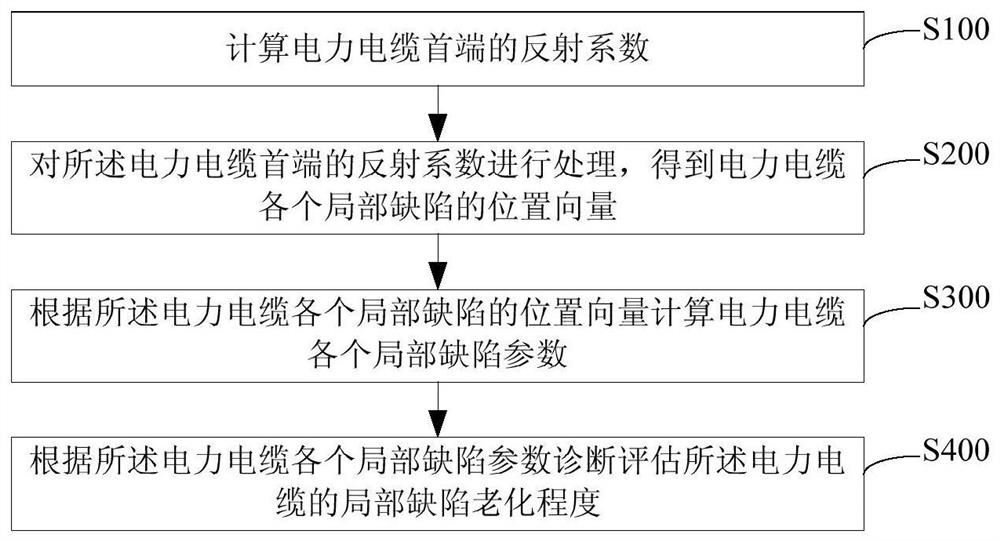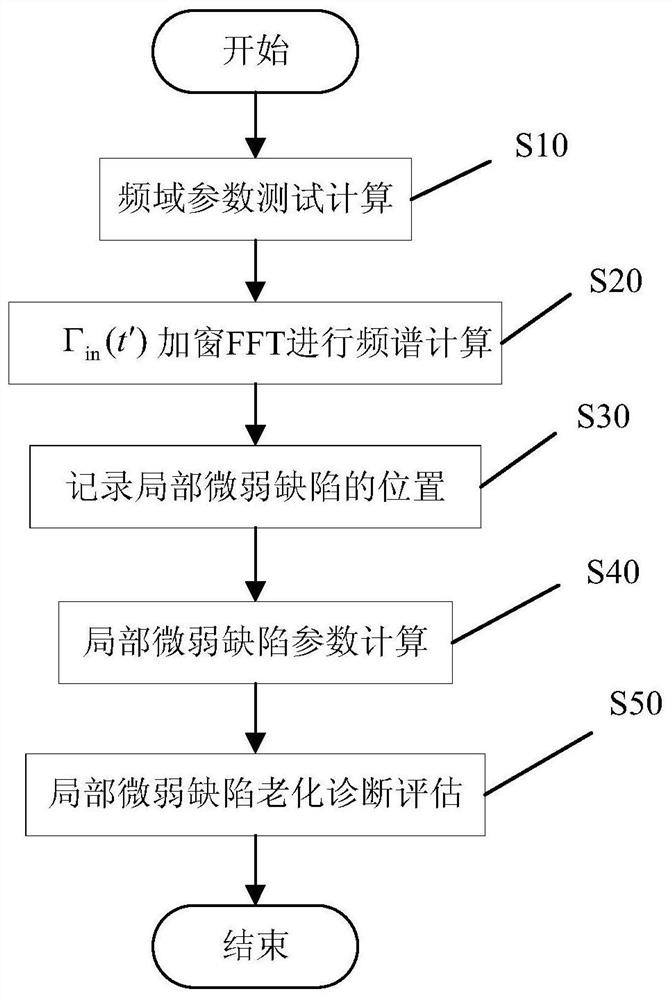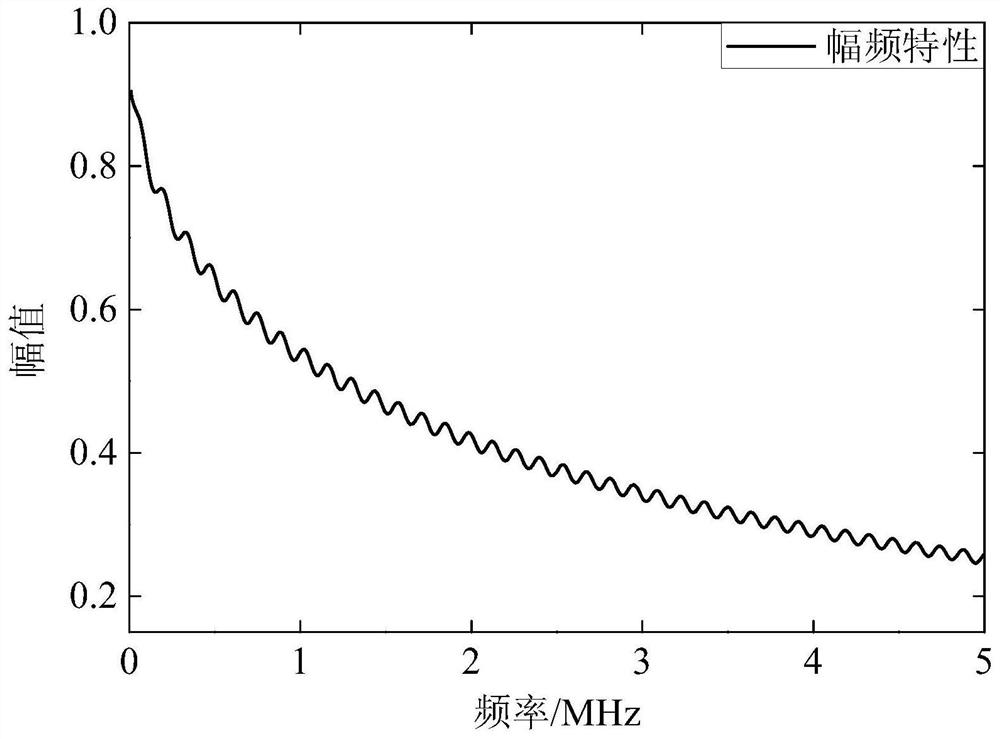# Local defect aging diagnosis and evaluation method for power cable

## A technology for local defects and power cables, applied in the direction of measuring resistance/reactance/impedance, measuring electrical variables, instruments, etc., to reduce the amount of calculation, reduce the difficulty of calculation, and improve the probability of successful calculation

Active Publication Date: 2021-08-20
JIANGSU ELECTRIC POWER CO
9 Cites 3 Cited by

## AI-Extracted Technical Summary

### Problems solved by technology

 The present invention provides a local defect aging diagnosis and evaluation method for power c...
View more

### Method used

By calculating the size of the absolute value of the reflection coefficient of different defect positions, the insulation aging degree of local weak defects has been effectively quantitatively judged;
 The optimization algorithm is used to calculate each local ...
View more

## Abstract

The invention relates to the technical field of power cables, and particularly discloses a local defect aging diagnosis method for a power cable, and the method comprises the steps: S100, calculating the reflection coefficient of the head end of the power cable; S200, processing the reflection coefficient of the head end of the power cable to obtain a position vector of each local defect of the power cable; S300, calculating each local defect parameter of the power cable according to the position vector of each local defect of the power cable; and S400, diagnosing and evaluating the local defect aging degree of the power cable according to each local defect parameter of the power cable. The local defect aging diagnosis method for the power cable provided by the invention can be used to simply and effectively diagnose local weak defect aging of the power cable.

Application Domain

Resistance/reactance/impedenceFault location

Technology Topic

Control theoryPower cable +1

## Image

•••## Examples

• Experimental program(2)

### Example Embodiment

 Example 1
 The measured power cable for the present embodiment is 1000M Zr-YJV02 8.7 / 15 3 * 95mm2 power cable, and the impedance is 1 kΩ in 300 m locations.
 This embodiment uses the Agilent E5061B vector network analyzer to test the heading input impedance of the test cable.
 This embodiment is provided for a local defective aging diagnosis method for a power cable, such as figure 2 As shown, including the following steps:
 S10, frequency domain parameter test calculation
 Setting the vector network analyzer is [10kHz, 5MHz], set the number of sweep points n to 1001 and input impedance Z in the first end of the power cable. in (f) Test, then calculate the reflection coefficient γ in the first end of the cable in (f):

 Calculated cable first end reflection coefficient γ in (f) image 3 Indicated. Where f is the scan frequency point; z 0 In order to use the same model, the same batch of 10M long reference cable measured the obtained characteristic impedance.
 S20, using conversion functions f → t 'will result in (f) Perform a conversion to obtain a T 'domain signal γ in (t '), then γ in (t ') Perform a gamma to the BLACKMAN window fast Fourier transform (FFT) in (t ') spectrum FFT [γ in (t ')] Figure 4 The spectrum absorbing is normalized (the figure is normalized).
 S30, observation Figure 4 Can know FFT [γ in (t ')] There is a position that can be characterized as partial weak defect point, and the local micro defect position is 300m.
 S40, partial weak defect parameters calculation
 S41, order to analyze local weak defect position L c 300M, then utilize formula γ ' in (f) = real (γ in (f)) · EXP (2α (f) L c ) Calculate reflection coefficient parameters γ ' in (f). Among them, REAL (·) is a complex real part; Exp (·) is an exponential function; α (f) is a decay constant measured by using the same model, the same batch of 10m long reference cable measurement.
 S42, using conversion functions f → t 'will result in γ' in (f) Convert to obtain T 'domain signal γ' in (t '), then γ' in (t ') performs γ' for the addition of Blackman window FFT processing in (t ') spectrum FFT [γ' in (t ')] Figure 5 The gray solid line is shown.
 S43, record FFT [γ ' in (t ')] China's weak defect position L c Signal intensity a c , Then use formula γ ' c1 (f) = γ ' in (f) -A c · COS (4πL c F / v (f)) / f w And γ ' c2 (f) = γ ' in (f) + a c · COS (4πL c F / v (f)) / f w Calculate γ ' c1 (f) and γ ' c2 (f). Where cos (·) is a cosine function; V (f) is the phase speed obtained by using the same model, the same number of 10m long reference cable measurements, F w Indicates the DC component size of the corresponding Blackman window function.
 S44, using conversion functions f → t 'will result in γ' c1 (f) and γ ' c2 (f) Convert to obtain T 'domain signal γ' c1 (t ') and γ' c2 (t '), then γ' c1 (t ') and γ' c2 (t ') is performed by adding Blackman window FFT processing to obtain γ' c1 (t ') and γ' c2 (t ') spectrum FFT [γ' c1 (T ')] and FFT [γ' c2 (t ')] Figure 5 The black solid line and gray dotted line are shown.
 S45, record FFT [γ ' c1 (T ')] and FFT [γ' c2 (t ')] Signal intensity A' at 300m in the middle local part c1 A ' c2 , Compare A ' c1 A ' c2 With a c the size of. Depend on Figure 5 Can know min {a ' c1 A ' c2 } = 0.07A c Max {a ' c1 A ' c2 } = 2A c Meet min {a ' c1 A ' c2 } ≤0.3a c And Max {a ' c1 A ' c2 } ≥1.7a c Therefore, the starting point of the defect is 300m, and the length of the defect is not 0m, and due to A ' c1 A ' c2 Therefore, the reflection coefficient of 300M position corresponds to defects ρ c = -A c / F w = -0.011. Where min (·) is the minimum; max (•) is the maximum.
 S50, local micro-defect aging diagnostic assessment
 Using the absolute value of the reflection coefficient to perform the local weak defects of different locations, the insulation aging state is evaluated. Since the partial weak defect reflection coefficient is 0.011, the defective aging state is medium.
 At the same time, due to the partial weak defect reflection coefficient ρ c = -A c / F w = -0.011 less than zero and the defect length is 0m, so the reflection of the local weak defect is negative, at which time the defect is a parallel impedance type defect or Z 0 Reduce type defects are the same as the actual defect type.
 In order to demonstrate the accuracy of the judgment, the cable is tested using the time domain reflection method (TDR) to obtain the test results. Image 6 Indicated. Depend on Image 6 It can be seen that there is a pulse waveform having a negative reflection polar in the 300m position in the TDR test result, whereby the above method can accurately determine the reflection polarity of the local weak defect of the cable.

### Example Embodiment

 Example 2
 The measured power cable for this embodiment is a 1000M Zr-YJV02 8.7 / 153 * 95 mm2 power cable, and there is a local weak defect in the 400m position.
 This embodiment uses the Agilent E5061B vector network analyzer to test the heading input impedance of the test cable.
 This embodiment is provided for a local defective aging diagnosis method for a power cable, such as figure 2 As shown, including the following steps:
 S10, frequency domain parameter test calculation
 Set the vector network analyzer's sweep range [10kHz], set the number of sweep points n to 1001 and input impedance Z in the first end of the cable in (f) Test, then calculate the reflection coefficient γ in the first end of the cable in (f):

 Where f is the scan frequency point; z 0 In order to use the same model, the same batch of 10M long reference cable measured the obtained characteristic impedance.
 S20, using conversion functions f → t 'will result in (f) Perform a conversion to obtain a T 'domain signal γ in (t '), then γ in (t ') Performing a BLACKMAN window fast Fourier transform (FFT) treatment to get γ in (t ') spectrum FFT [γ in (t ')].
 S30, from γ in (t ') spectrum FFT [γ in (t ')] It can be seen that there is a position that can be characterized as a local micro defect point, and the local weak defect position is 400.4711 m.
 S40, partial weak defect parameters calculation
 S41, order to analyze local weak defect position L c Take 400.4711m, then utilize formula γ ' in (f) = real (γ in (f)) · EXP (2α (f) L c ) Calculate reflection coefficient parameters γ ' in (f). Among them; REAL (·) is a plurality of real part; Exp (·) is an index function; α (f) is the attenuation constant obtained by using the same model, the same batch of 10m long reference cable measurement.
 S42, using conversion functions f → t 'will result in γ' in (f) Convert to obtain T 'domain signal γ' in (t '), then γ' in (t ') performs γ' for the addition of Blackman window FFT processing in (t ') spectrum FFT [γ' in (t ')] Figure 7 The gray solid line is shown.
 S43, record FFT [γ ' in (t ')] China's weak defect position L c Signal intensity a c , Then use formula γ ' c1 (f) = γ ' in (f) -A c · COS (4πL c F / v (f)) / f w And γ ' c2 (f) = γ ' in (f) + a c · COS (4πL c F / v (f)) / f w Calculate γ ' c1 (f) and γ ' c2 (f). Where cos (·) is a cosine function; V (f) is the phase speed obtained by using the same model, the same number of 10m long reference cable measurements, F w Indicates the DC component size of the corresponding window function.
 S44, using conversion functions f → t 'will result in γ' c1 (f) and γ ' c2 (f) Convert to obtain T 'domain signal γ' c1 (t ') and γ' c2 (t '), then γ' c1 (t ') and γ' c2 (t ') is performed by adding Blackman window FFT processing to obtain γ' c1 (t ') and γ' c2 (t ') spectrum FFT [γ' c1 (T ')] and FFT [γ' c2 (t ')] Figure 7 The black solid line and gray dotted line are shown.
 S45, record FFT [γ ' c1 (T ')] and FFT [γ' c2 (t ')] Signal intensity a' at a micro-defect position 400.4711m c1 A ' c2 , Compare A ' c1 A ' c2 With a c the size of. Depend on Figure 7 Can know min {a ' c1 A ' c2 } = 1.43A c Max {a ' c1 A ' c2 } = 1.46A c Not satisfying min {a ' c1 A ' c2 } ≤0.3a c And Max {a ' c1 A ' c2 } ≥1.7a c. Where min (·) is the minimum; max (•) is the maximum.
 S46, assuming that the local weak defect length is 1m, let G x (l x 1M, ρ x ) | f for:
 G x (l x 1M, ρ x ) | f = Γ ' in (f) -ρ x · COS (4πL x F / v (f)) + ρ x · (1-ρ x 2 ) · COS (4π (L) x +1) · f / v (f)))
 Where L x To be optimized, the local weak defect start position, the value range is [384.4711m, 416.4711M]; ρ x For the preferred reflection coefficient, the value range is [-0.5, 0.5].
 S47, using conversion functions f → t 'will get G x (l x 1M, ρ x ) | f Convert to get the T 'domain signal G x (l x 1M, ρ x ) | t′ , Then use intelligent optimization algorithms to L x Ρ x Perform G x (l x 1M, ρ x ) | t′ The global optimization of the signal amplitude at 400.4711m after the BLACKMAN window FFT was added, and the starting position and reflection coefficient of the local micro-defect at 400.4711 m were solved were 399.9974 m and -0.05, respectively.
 S50, local micro-defect aging diagnostic assessment
 Using the absolute value of the reflection coefficient to perform an insulating aging state of the partial weak defects of different locations, since the partial weak defect reflection coefficient is 0.05, the defective aging state is medium.
 At the same time, since the local weak defect reflection coefficient is less than zero and the defect length is 1m, the reflection at the local weak defect is negative, and the defect is Z 0 Reduce type defects.
 In order to prove the accuracy of the judgment result, the cable is tested using TDR to obtain the test results. Figure 8 Indicated. Depend on Figure 8 It can be seen that there is a pulse waveform of the 400m in the TDR test result in the first negative positive pulse waveform, whereby the above method can accurately determine the reflection polarity of the local weak defect of the cable.

## PUM## Description & Claims & Application Information

We can also present the details of the Description, Claims and Application information to help users get a comprehensive understanding of the technical details of the patent, such as background art, summary of invention, brief description of drawings, description of embodiments, and other original content. On the other hand, users can also determine the specific scope of protection of the technology through the list of claims; as well as understand the changes in the life cycle of the technology with the presentation of the patent timeline. Login to view more.

## Kinect depth image-based human body fall detection method and device

Owner:NANCHANG UNIV

## Network data flow recognizing system and method

InactiveCN1852297Asmall amount of calculation
Owner:江苏钊阳冷暖科技有限公司

Owner:重庆海睿科技有限公司

## Fundamental wave and harmonic wave detecting method based on three-coefficient Nuttall windowed interpolation FFT

InactiveCN101701984Asmall amount of calculationImprove operational stability
Owner:ZHEJIANG UNIV

## Image super-resolution reconstruction method based on multi-column convolution neural network

PendingCN109255755Asmall amount of calculationImprove rebuild speed
Owner:SHANGHAI UNIV

## Classification and recommendation of technical efficacy words

• small amount of calculation
• Reduce computational difficulty

## License plate detection method based on deep learning

InactiveCN106022232ARapid detectionsmall amount of calculation
Owner:CHENGDU XINEDGE TECH

## Unmanned vehicle real-time positioning method based on laser reflection strength

Owner:TONGJI UNIV

## Vehicle path planning method based on storage unmanned vehicle

InactiveCN107037812AOptimizing Topology Nodessmall amount of calculation
Owner:NANJING UNIV OF SCI & TECH

## Phase interferometer direction finding method for ambiguity resolution by extension baselines

InactiveCN102411136AImprove direction finding accuracysmall amount of calculation
Owner:UNIV OF ELECTRONIC SCI & TECH OF CHINA

## Motion noise interference eliminating method suitable for wearable heart rate monitoring device

InactiveCN104161505AHigh removal accuracysmall amount of calculation
Owner:BEIJING UNIV OF POSTS & TELECOMM
Who we serve
• R&D Engineer
• R&D Manager
• IP Professional
Why Eureka